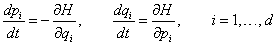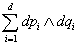## Numerical Hamiltonian Problems

Hamiltonian systems, given bywhere H(p,q) a Hamiltonian function and d is the number of degrees of freedom of the system can be seen to possess two remarkable properties:

• The solutions preserve the Hamiltonian H(p,q), i.e., H=Constant
• The corresponding flow is sympletic, i.e., preserves the differential 2-form. For d=1, this corresponds to preservation of area.

Both of the above properties are usually destroyed by numerical methods applied to Hamiltonian systems. A class of numerical methods, referred to as sympletic methods, do produce numerical solutions with the above mentioned two properties. Sympletic Integration methods are the key to the successful long time integration of Hamiltonian Systems.

The image on this page is the exact solution to Kepler's Orbit problem in black, with the corresponding numerical solution produced by a non-sympletic fourth order explicit Runge-Kutta method. A fourth order sympletic method will produce dramatically different results.

The Applet demonstrates the integration of six model Hamiltonian systems by a variety of sympletic and non-sympletic methods. The methods are listed below with their order of accuracy in parentheses and with * denoting that the methods is sympletic.

Explicit Methods: Euler(1), Improved Euler(2), Runge-Kutta(3), "Classical" Runge-Kutta(4), Runge-Kutta(6), Stormer-Verlet Runge-Kutta-Nystrom(2)*, Calvo Runge-Kutta-Nystrom(4)*

Implicit Methods: Implicit Euler(1), Trapezoid(2), Midpoint(2)*, Gauss(4)*, Gauss(6)*, Gauss(8)*, RadauIA(5)

Problems: Harmonic Oscillator, Pendulum, Double Harmonic Oscillator, Kepler's Problem, Modified Kepler Problem, Henon-Heiles

The Examples problems are from the book Numerical Hamiltonian Problems, J.M. Sanz-Serna and M.P. Calvo, Chapman & Hall, 1994.